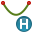# Hyperbolic Arc

Create a hyperbolic arc to be used either as a building block for constructing geometry or as a wire.

Hyperbolic arcs are often used in conjunction with the spin operator to create hyperbolic dishes for reflector antennas.

On the Construct tab, in the Create Arc group, click theHyperbolic Arc icon.
Tip: Press A,3 to use the shortcut key.

## Method 1: Base centre, depth, radius, eccentricityBase centre (C) The centre of the hyperbola on which the arc lies. Depth (D) The distance from the apex of the hyperbola to the centre of the arc aperture. Radius (R) The radius of the aperture of the hyperbolic arc. Eccentricity The eccentricity of the hyperbola on which the hyperbolic arc section lies.
Conditions to create a valid hyperbolic arc:
(1) $1\le \epsilon \le \sqrt{1+\frac{{R}^{2}}{{D}^{2}}}$
where D is denoted by the depth, R by the aperture radius R and $\epsilon$ the eccentricity.

## Method 2: Aperture centre, depth, radius, eccentricityAperture centre (C) The centre of the aperture formed by the hyperbolic arc. Depth (D) The distance from the apex of the hyperbola to the centre of the arc aperture. Radius (R) The radius of the aperture of the hyperbolic arc. Eccentricity The eccentricity of the hyperbola on which the hyperbolic arc section lies. The eccentricity must be greater than one to specify a valid hyperbola.Note: Not all values greater than one specifies a valid hyperbola.
Conditions to create a valid hyperbolic arc:
(2) $1\le \epsilon \le \sqrt{1+\frac{{R}^{2}}{{D}^{2}}}$
where D is denoted by the depth, R by the aperture radius R and $\epsilon$ the eccentricity.